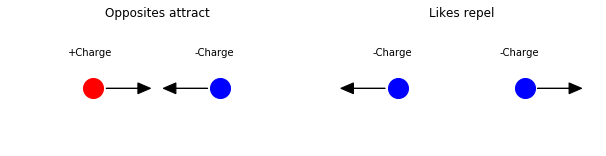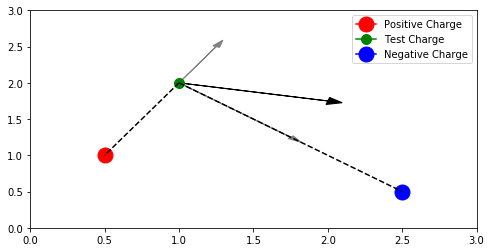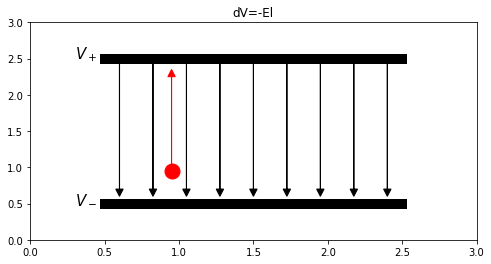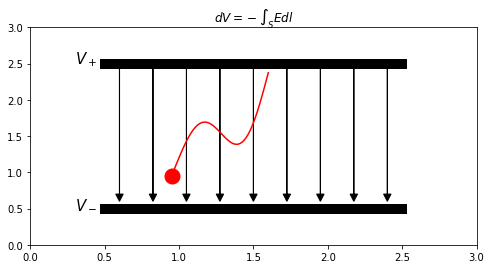Notebook

# Electromagnetic Fields

In :
% run emf_functions.py


The code used in this chapter can be found here.

## Chapter 1: Electric Fields

### Coloumb's Law

Protons attract electrons but repulse other protons. Electrons attract protons but repulse other electrons. The bigger the proton or electron, the stronger this force is. The bigger the distance between them, the weaker this force is. In other words, the magnitude of attraction or repulsion between the two point charges is proportional to the magnitudes of the charges and inversely proportional to the square of the distance between them. This is shown in the formula, $F = k_e.\cfrac{Q_1.Q_2}{r^2}=\cfrac{Q_1.Q_2}{4.\pi.\varepsilon.r^2}$, where $k_e$ is Coloumb's constant and $\varepsilon$ is the permittivity of the medium.

In :
coloumb_example()### Test Charge

The electric field is defined as the force any +1C charge would experience if it was placed at a any location. Therefore this force is defined as $\vec{F}=e.\vec{E}$, where $E$ is the electric field. To measure this field, an imaginary test charge with magnitude 1 is placed at imaginary location. Then $E=\cfrac{q}{4.\pi.\varepsilon.r^2}$. The electric field can be then drawn as a vector at each point of the field. The unit used for electric fields is Siemens.

In :
test_charge_example(x0 = 0.5, y0 = 1,
x1 = 2.5, y1 = 0.5,
x2 = 1,   y2 = 2)### Relation to Voltage

Since the voltage is the work done per charge and word done is equal to force x distance, voltage is a product of the force per charge (aka. Electric field) and the distance being measured, $V=E.l$. However, that formula applies only for the simplest case when the field is going alone a straight electric field, such as the example below:

In :
line_charge_example1()To represent a charge that goes along an arbitrary 3D path S, the formula can be generalized to $dV=\int_S\vec{E}.d\vec{l}$ or $E = -dV/dS = -(\cfrac{dv}{dx}.\hat{i}+\cfrac{dv}{dy}.\hat{j}+\cfrac{dv}{dz}.\hat{k})=-\triangledown.V$.

(Check out partial derivatives to understand this equation.)

In :
line_charge_example2()### Kirchoff's Voltage Law

However, the last formulation shows that the path that the charge takes is irrelevant. Any path between points A and B will produce the same result for $dV=V_A-V_B$. Further analysing this, a path from A to A produces $dV=V_A-V_A=0$, therefore any path that is closed would produce a net voltage of 0.

### Equipotential

Adjacent points that has the same voltage can be called equipotential. Any charge moving along lines of equipotential points, does no work because there is no change in voltage potential. Because there is no change in potential, there is no electric field along equipotentail lines.

### Ideal Conductors

Ideal conductors are equipotential because they have no resistance. Therefore the electric field inside ideal conductors is zero. Electric field lines are perpendicular to equipotential lines. Because of that any charge within the conductor must be at the surface.